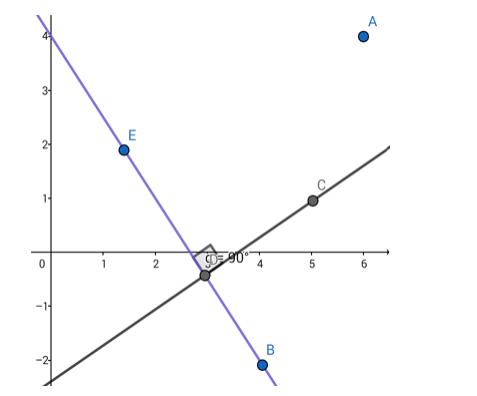# Find the equation of the line which is perpendicular to the lineQuestion:

Find the equation of the line which is perpendicular to the line 3x + 2y = 8 and passes through the midpoint of the line joining the points (6, 4) and (4, - 2).

Solution:Given: The given line is $3 x+2 y=8$. The perpendicular line passes through the midpoint of $(6,4)$ and $(4,-2)$.

Formulae to be used: The product of slopes of two perpendicular lines $=-1$.

If (a,b) and (c,d) be two points, then their midpoint is given by

$\left(\frac{a+c}{2}, \frac{b+d}{2}\right)$

The slope of this line is $-3 / 2$.

∴ the slope of the perpendicular line =

$\frac{-1}{-3 / 2}=2 / 3$

The equation of the line can be written in the form

$y=\left(\frac{2}{3}\right) x+c$

(c is the $y$ - intercept)

This line passes through the midpoint of $(6,4)$ and $(4,-2)$.

The co - ordinates of the midpoint of the line joining the given points is

$\left(\frac{6+4}{2}, \frac{4+(-2)}{2}\right)=(5,1)$

(5,1) satisfies the equation

$y=\left(\frac{2}{3}\right) x+c$

$\therefore 1=\left(\frac{2}{3}\right) \times 5+\mathrm{c}$ or, $\mathrm{c}=1-\frac{10}{3}=-\frac{7}{3}$

The required equation is

$y=\left(\frac{2}{3}\right) x+\left(-\frac{7}{3}\right)$

i.e. $2 x-3 y=7$